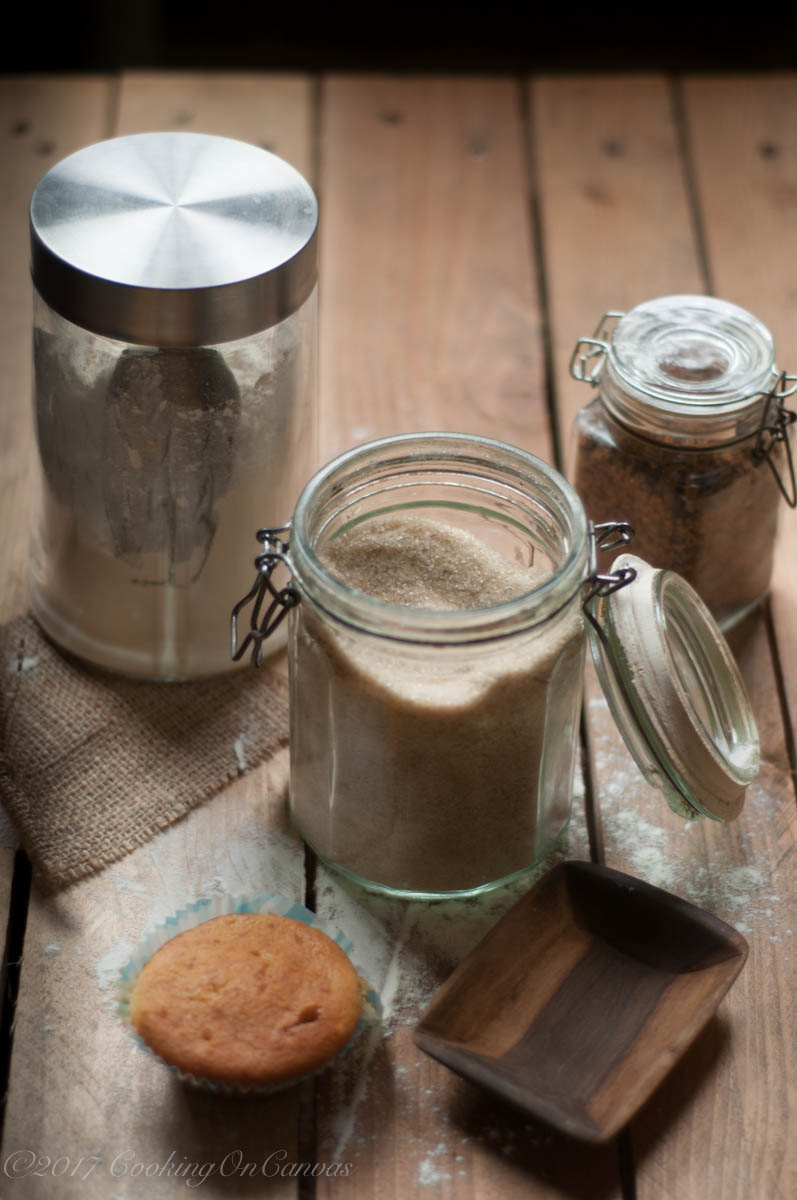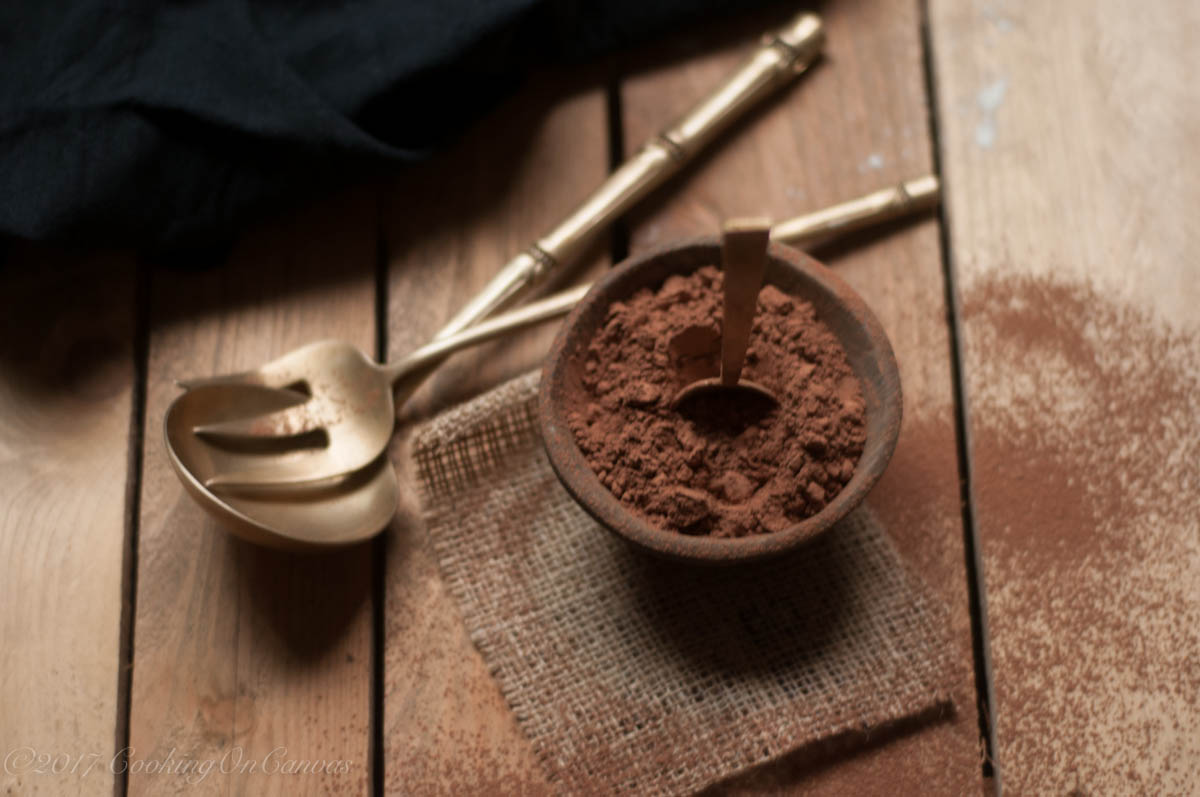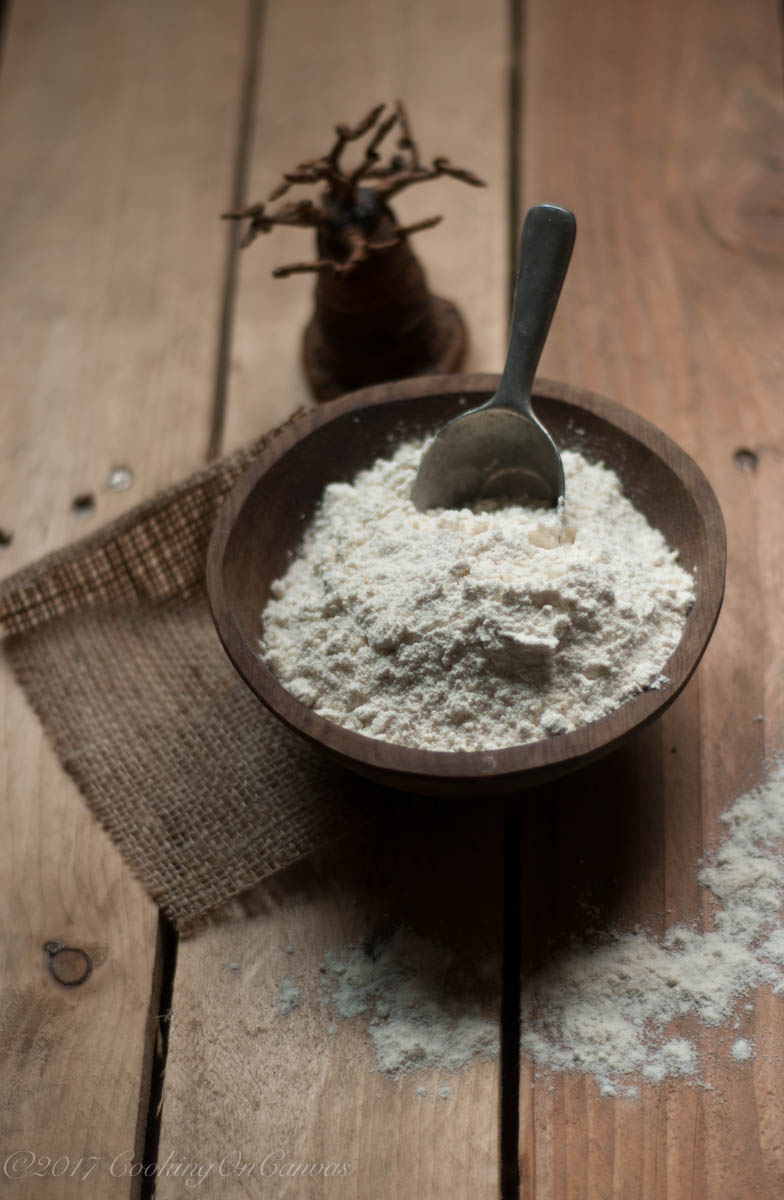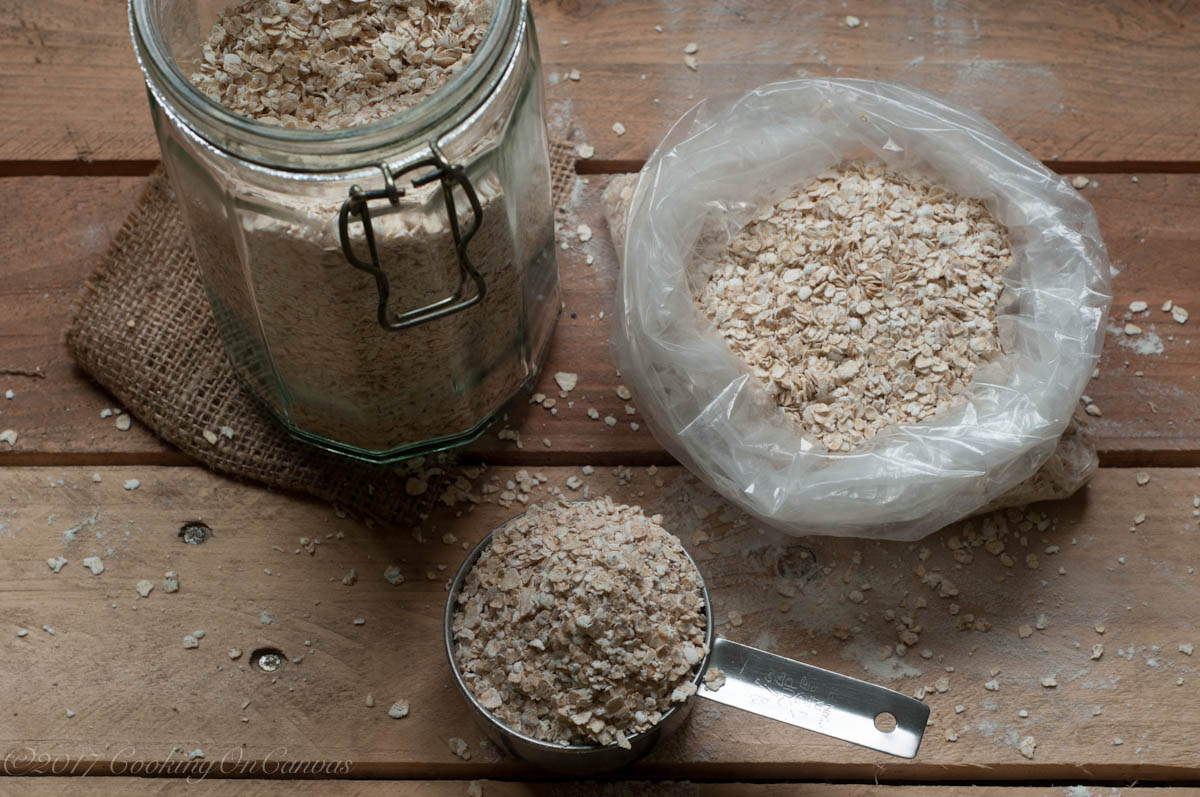# ﻿Conversion

It seems to happen that just after I have greased a tin or started kneading, that will be the moment I need to reach for my phone to convert the weights in a recipe. The majority of the time though, the home button—actually my phone in general—tends to be covered with flour. It is no surprise that on my wish list is Google Home.But since that is probably not going to happen, the next best thing I figured would be to combine in one place most of ingredients I am constantly converting from volume to weight or vice versa. Just for the record, volume is way easier but weight is more accurate.  I prefer measuring by weight when it comes to breads and pastry but volume for cakes and cookies.

Celcius to Fahrenheit
220C =425F
205C = 400F
190C = 375F
180C = 350F
160C = 325F
150C = 300F
135C = 275F
120C = 250F
107C = 225FAll-Purpose Flour
1 cup = 125 grams
3/4 cup =93.75 grams
2/3 cup = 83 grams
1/2 cup = 62.5 grams
1/3 cup = 42 grams
1/4 cup = 31.25 grams
1 Tablespoon = 9.38 gramsSugar
1 cup = 200 grams
3/4 cup = 150 grams
2/3 cup = 134 grams
1/2 cup = 100 grams
1/3 cup = 67 grams
1/4 cup = 50 grams
2 Tablespoons = 25 grams
1 Tablespoon = 12.5 gramsCocoa
1 cup = 105 grams
3/4 cup = 80 grams
2/3 cup = 70 grams
1/2 cup = 55 grams
1/3 cup = 35 grams
1/4 cup = 25 grams
1 Tablespoon = 7 gramsCornflour
1 cup = 120 grams
3/4 cup = 90 grams
2/3 cup = 80 grams
1/2 cup = 60 grams
1/3 cup = 40 grams
1/4 cup = 30 grams
2 Tablespoons = 15 gramsOats
1 cup = 85 grams = 3oz
3/4 cup = 63 grams
2/3 cup = 57 grams
1/2 cup = 43 grams
1/3 cup = 28 grams
1/4 cup = 21 grams
2 Tablespoons = 11 grams

Yeast
3.5 grams = 1 teaspoon
1 package yeast = 7 grams = 2 1/4 teaspoons

Butter
1 cup = 226 grams = 2 sticks = 8 ounces
200 grams = 14 Tablespoons
1/2 cup = 113 grams
1/3 cup = 76 grams
1/4 cup = 57 grams

Shortening
1 cup = 190 grams
3/4 cup = 145 grams
2/3 cup = 125 grams
1/2 cup = 95 grams
1/3 cup =65 grams
1/4 cup = 45 grams

Milk
1 pint = 500 ml = 2 cups
1 cup = 250ml
3/4 cup = 187.5 ml
1/2 cup = 125 ml
1/4 cup = 62.5 ml = 4 Tablespoons

1.Rita says:

Thank you, this is so useful.

1.cookingoncanvas says:

Karibu!

2.Jeni says:

Always knew you were a smart cookie 😘

1.cookingoncanvas says:

Thank you 😊 . Although it was born of necessity 😘

3.Bybron says: